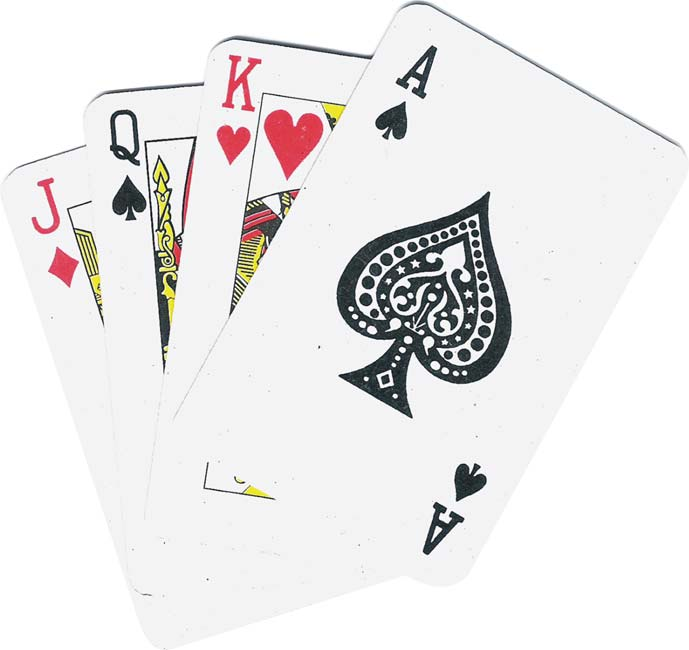Back by popular demand is my most popular post of all time, original linked here: http://farrellink.com/2009/09/10/tutor-tip-forget-flash-cards-try-math-war/

Use an ordinary deck of playing cards to relax with math. Try Math War to practice basic math operations:1. Take out the Jokers, Kings, 10s, and Queens.
2. Deal the remaining deck between two players.
3. One rule: Aces are One, and Jacks are Zero.
4. Each player flips up a card and the student has to add, subtract, multiply or divide the numbers.
5. When the student is right, he or she keeps the cards until the whole deck has been won!

You can use this method of playing cards to practice any type of math, all the way to the high school or GED level and beyond. This includes algebra and geometry! But how, you ask? First of all, you will need to grab a pencil and paper for these brain workouts.  I suggest starting with a round of the lighter stuff before getting started on the heavy lifting. Because of the element of chance, these problems often don’t work out to have easier answers…just like numbers in real life.

1. More numbers: each player flips up more than one card at a time.
2. Mixed practice: flip up a card and the tutor randomly chooses between add, subtract, multiply, or divide.
3. More mixed practice: Take a blank piece of scrap paper and fold it in half 4 times. Unfold the paper and cut on the lines to make 16 pieces. Make one of the operation signs (+ – x ./.) on 4 pieces each.
4. Fractions: one card (or more) is the numerator and one card (or more) is the denominator.
5. Decimals: use a face card or a piece of scrap paper to make the decimal point.
6. Percents & ratios: use a face card or piece of scrap paper to make the decimal point.
7. Algebra: add the face cards back in to become your variables. You might also want to make pieces of scrap paper with (parentheses), square roots, and positive or negative signs.
8. Geometry: start with your formulas and use the flash cards to get the numbers.

LOL FUN: Return to the simplest version of Math War. When each player flips up a card, make a real life word problem using the numbers. And since this article is meant for adult literacy tutors, you don’t have to censor the results. Trust me, this will hold your students’ interest and make math much less intimidating!